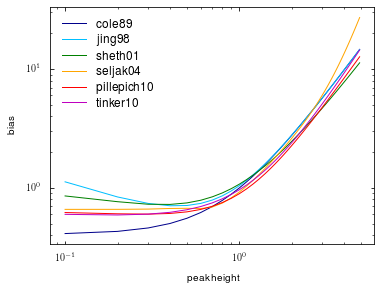# Colossus tutorial: Halo bias¶

Welcome to the Colossus halo bias tutorial.

In :
from __future__ import print_function
import matplotlib.pyplot as plt
import numpy as np
%matplotlib inline


We begin by setting a cosmology:

In :
from colossus.cosmology import cosmology
cosmology.setCosmology('planck15');


Halo bias quantifies the excess clustering of halos over the clustering of dark matter. Bias is, in general, a function of halo mass and spatial scale, but this module implements only scale-free bias models. Moreover, one can apply higher-order bias corrections but this module is restricted to linear bias. Let's make a plot of the predictions of the different models:

In :
from colossus.lss import bias

z = 0.0
mdef = '200m'
nu = np.arange(0.1, 5.0, 0.1)

plt.figure()
plt.xlabel('peak height')
plt.ylabel('bias')
plt.loglog()
for model in bias.models:
bias_model = bias.haloBiasFromNu(nu, z, mdef, model = model)
plt.plot(nu, bias_model, '-', label = model)
plt.legend();